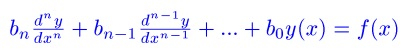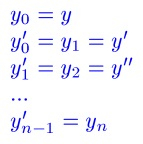Friday, September 13, 2013

Differential Equations #12: Reduction of Order

Introduction

The general n-order differential equationcan be translated into a system of first-order differential equations:For a second-order equation

y" + A*y' + B*y = f(x)

The system is

y'_1 = y_2
y'_2 = f(x) - A*y_2 - B*y_1

Now time for examples.

1. y" + 3*y' - 4*y = 2

Let y_1 = y and y_2 = y'_1. Then:
y_2 = y', and

y'_2 = y"
y'_2 = 2 - 3*y' - 4*y
= 2 - 3*y_2 - 4*y_1

So our system is:
y'_1 = y_2
y'_2 = 2 - 3 * y_2 - 4 * y_1

2. 3*y" + 6*y' = -9

Let y_1 = y and y_2 = y'. Then:

y'_1 = y_2
y'_2 = y"
3*y" = -9 - 6*y'
y" = -3 - 2*y'
y'_2 = -3 - 2*y_2

The system is:
y'_1 = y_2
y'_2 = -3 - 2*y_2

We can go the other way, from a system of linear differential equations to a single multi-order differential equation.

3. y'_1 = y_2
y'_2 = y_1 + y_2

Again, let y_1 = y and y_2 = y'. Then:
y'_2 = y_1 + y_2
y" = y + y'
y" - y' - y = 0

4. y'_1 = y_2
y'_2 = 2/7 * y_1 - 3/7 * y_2 + 1/7

With y_1 = y and y_2 = y',
y'_2 = 2/7 * y_1 - 3/7 * y_2 + 1/7
y" = 2/7 * y - 3/7 * y' + 1/7
y" + 3/7 * y' - 2/7 * y = 1/7
7*y" + 3*y' - 2*y = 1

This concludes this blog post of Differential Equations. In a week I will be headed to Fort Collins. CO for the HHC 2013 conference. I am so excited - may the bad weather go away and everyone be safe!

Eddie

This blog is property of Edward Shore. 2013